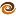# EmptyCrate

#### About Me | Contact Me | Training |ChaiScript.com | YouTube Channel

Last week I noticed that the MySQL “sounds like” operator uses the soundex algorithm to determine if two words sound alike. The algorithm is English centric and was designed around the time of the time of the 1880 census. It has been used in the US census since 1880 to account for miss or different spellings of family names.

According to Knuth, the algorithm is also used in airline reservation systems to account for reservation spelling errors.

It seems the algorithm rose to prominence around the 1960’s when the Communications of the ACM ran a series of articles discussing it and Knuth covered it in his Art of Computer Programming. Wikipedia has some information about it but the algorithm they describe is very poorly worded. A better treatment of it is available at the creativyst.

The algorithm takes a word and converts it to a number. It fascinates me. Not specifically because it can approximate if two words sound the same but more because it raises an important question that is applicable to all manner of information fields: “How much information can you discard and still have something meaningful?” This is a question that any lossy compression (video, graphic or audio) asks. It’s also a question that needs to be asked when encoding things like DNA samples or fingerprints. We should ask this question anytime we have to analyze a dataset with inherent error in it and we need to normalize against this error.

I’m fairly certain I’ll be able to use a technique similar to this to fingerprint hosts on a network to determine if two “different” hosts collected by two different means are actually the same host.

I’ve implemented soundex in Haskell (below). My implementation follows these steps:

1. Convert all chars after the first character to lower case
2. Convert characters to their numerical mappings:

f, p, v -> 1 c, g, j, k, q, s, x, z -> 2 d ,t -> 3 l -> 4 m, n -> 5 r -> 6

3. Remove any ‘H’ or ‘W’ that is between two numbers that are the same
4. Remove all repeating numbers
5. Remove any non-number
6. Append the previously dropped first character
7. Drop all non-numbers after the first one

The classic examples are names like “Rupert” and “Robert” both generating the code “R163.”

``````import Data.Char (toLower, toUpper, isNumber)
charToInt :: Char -> Char

charToInt x |    x == 'b'
|| x == 'f'
|| x == 'p'
|| x == 'v'    = '1'
|    x == 'c'
|| x == 'g'
|| x == 'j'
|| x == 'k'
|| x == 'q'
|| x == 's'
|| x == 'x'
|| x == 'z'    = '2'
|    x == 'd'
|| x == 't'    = '3'
|    x == 'l'    = '4'
|    x == 'm'
|| x == 'n'    = '5'
|    x == 'r'    = '6'
| otherwise = x

removeDups :: (Eq a)=>[a]->[a]
removeDups (x:x2:xs) | x == x2   = removeDups (x2:xs)
| otherwise = x : removeDups (x2:xs)

removeDups x       = x

removeNonNums (x:xs) | isNumber(x)  = (x:removeNonNums xs)
| otherwise = removeNonNums xs

removeNonNums _ = []

handleHW (x1:x2:x3:xs) | x1 == x3
&& (x2 == 'w' || x2 == 'h') = x1:handleHW (x3:xs)
| otherwise = x1:handleHW(x2:x3:xs)

handleHW x         = x

soundex (x:xs) = take 4 (( (toUpper x):removeNonNums (removeDups (handleHW (map charToInt (map toLower xs)))) )++"0000")`
``````